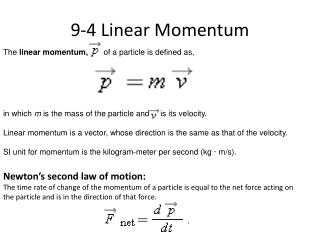DownloadDownload Presentation9-4 Linear Momentum

# 9-4 Linear Momentum

Télécharger la présentation## 9-4 Linear Momentum

- - - - - - - - - - - - - - - - - - - - - - - - - - - E N D - - - - - - - - - - - - - - - - - - - - - - - - - - -
##### Presentation Transcript

1. 9-4 Linear Momentum The linear momentum, of a particle is defined as, in which m is the mass of the particle and is its velocity. Linear momentum is a vector, whose direction is the same as that of the velocity. SI unit for momentum is the kilogram-meter per second (kg · m/s). Newton’s second law of motion: The time rate of change of the momentum of a particle is equal to the net force acting on the particle and is in the direction of that force.

2. 9-5 The Linear Momentum of a System of Particles The linear momentum of a system of particles is equal to the product of the total mass M of the system and the velocity of the center of mass.

3. 9-6 Collision and Impulse, Problem 26:In a common but dangerous prank, a chair is pulled away as a person is moving downward to sit on it, causing the victim to land hard on the floor. Suppose the victim falls by 0.50 m, the mass that moves downward is 70 kg, and the collision on the floor lasts 0.082 s. What are the magnitudes of the (a) impulse and (b) average force acting on the victim from the floor during the collision?

4. 9-7 Conservation of Linear Momentum If no net external force acts on a system of particles, the total linear momentum of the system cannot change. If the component of the net external force on a closed system is zero along an axis, then the component of the linear momentum of the system along that axis cannot change. Problem 39: A 91 kg man lying on a surface of negligible friction shoves a 68 g stone away from himself, giving it a speed of 4.0 m/s. What speed does the man acquire as a result?